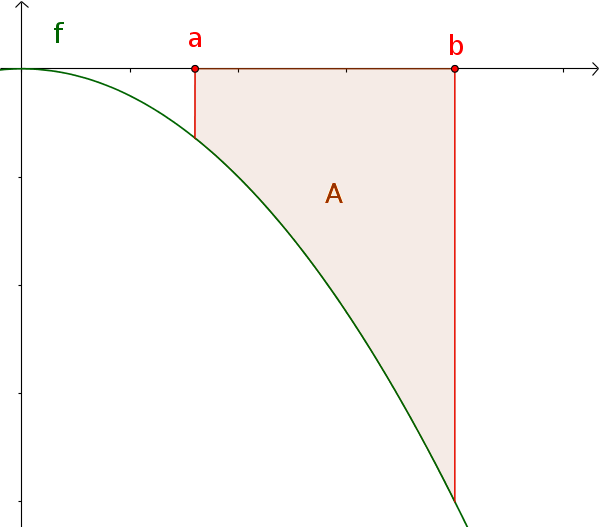Math Application integral calculus Calculating areas with integrals

# Area under a curve

The area between a curve and the x-axis can be calculated with the definite integral.

Here is a short overview. You can find more information about calculating areas with integrals in the corresponding article.

!

### Remember

For the definite integral, the partitions above the x-axis are positive and those below, negative.

## Functions without change of sign

The area under a function $f(x)$ without change of sign over $[a; b]$ corresponds to the absolute value of the definite integral.

$A=|\int_a^b f(x)\,\mathrm{d}x|$## Functions with change of sign

The area is partly above and partly below the x-axis. The divided areas must therefore be calculated and added separately.

$A=A_1+A_2$## Area between curves

The enclosed area in the interval $[a; b]$ is calculated by the formula:

$A=|\int_a^b (f(x)-g(x))\,\mathrm{d}x|$